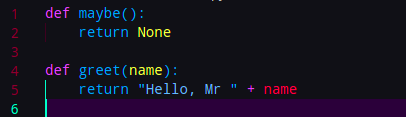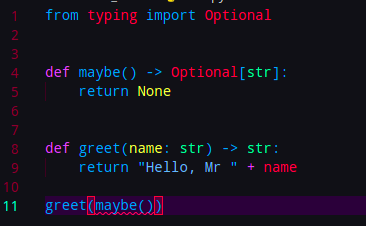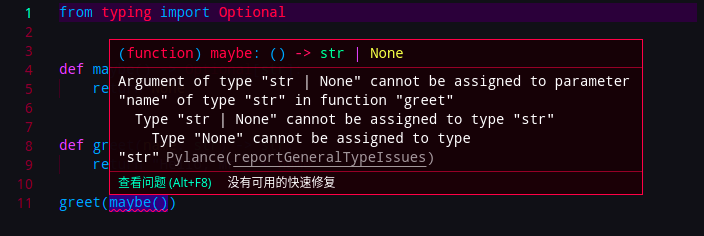# 探究Python类型注解

## 类型注解

Python是一门动态强类型语言，动态类型语言的特点就是灵活，比如在Java中有一种多态形式叫函数重载，多个函数可以有相同的名字，但是参数数量和类型不同，但是在Python中，函数参数的类型是动态的，数量也可以是动态的：

``````def add(lhs, rhs):
return lhs + rhs

def init(*args, **kwargs):
pass
``````## dataclass

``````class A:
greet = staticmethod(greet)

# 不会给出提示
A.greet(12)
``````

``````# person加注解和不加，结果不同
def hof(person):
greet(person.name)
``````

``````class Foo:
@classmethod
def create(cls, *args) -> Foo:
...
``````

`Python3.7`带来了一个新功能，叫做`Data Class`，如下代码：

``````from dataclasses import dataclass

@dataclass
class Point:
x: float
y: float
``````

``````from django.db import models

class Person(models.Model):
first_name = models.CharField(max_length=30)
last_name = models.CharField(max_length=30)
``````

PyCharm understands @dataclass decorator, but doesn't understand your custom decorator @mydataclass. You could use @dataclass for MyTest and change it to be similar to Test.

If it is required to have custom decorators this way, then the only option is to write some plugin for PyCharm.

## TypedDict

Python中的函数有着不定长度的可变参数定义：

``````def foo(*args, **kwargs):
...
``````

``````foo(a, b, c, d, e=1, f=2, g=3)
``````

``````class Point2D(TypedDict):
x: int
y: int
label: str

a: Point2D = {'x': 1, 'y': 2, 'label': 'good'}  # OK
b: Point2D = {'z': 3, 'label': 'bad'}           # Fails type check

assert Point2D(x=1, y=2, label='first') == dict(x=1, y=2, label='first')
``````

``````def foo(*args: *Args, **kwargs: **KArgs): ...
``````

EOF

Copyright © 2020-2021 公子政的宅日常